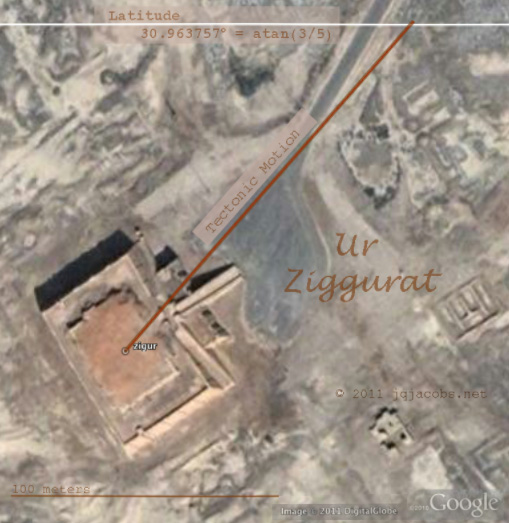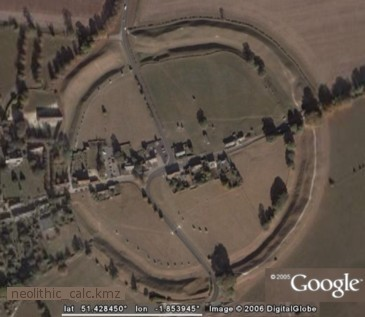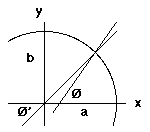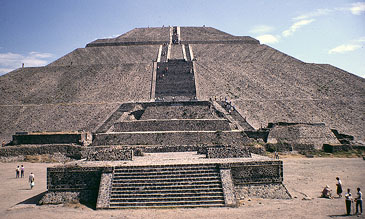GEODESY PAGE by James Q. JacobsGoogle Earth™ Ancient Monuments Placemarks Mounds, Pyramids, Stone Circles, more. DEFINITIONS: Geodesy is the discipline that deals with the measurement and representation of the earth, its gravity field and geodynamic phenomena (polar motion, earth tides, and crustal motion) in three-dimensional time varying space. Geodesy is primarily concerned with positioning and the gravity field and geometrical aspects of their temporal variations. Geoid is essentially the real shape of the earth, without accounting for the topographic features. It is an idealized equilibrium surface. The geoid, unlike the ellipsoid, is too complicated to serve as the computational surface on which to solve geometrical problems like point position. Coordinate System. A reference system for defining points in space or on planes and surfaces with relation to designated axis, planes or surfaces. In surveying and mapping three general types are used: (1.) Plano-polar, in which points in a plane are defined by distance from a specified point along a ray having a specified direction with respect to a base line; (2.) Rectangular, points are defined by distance from two perpendicular axis or three mutually perpendicular planes; (3.) Spherical, points on a surface are defined by the angles between a normal or radius through a point and two perpendicular diametrical planes. Geocentric Latitude (Ø') is defined by the angle between the equatorial plane and a line from the local position to the intersection of the axis of rotation with the equatorial plane. Determination based on a spherical earth is also termed Authalic Latitude. Geodetic Latitude (Ø) is defined by the angle between the plane of the geodetic equator and the local direction of the geodetic vertical. Geodetic Latitude and Geographic Latitude are practically the same. Geographic Latitude is obtained by surface observation of angular altitude of the visible pole. Due to flattening of the geoellipsoid, local plumb variates from geocentric in relation to distance from the equator and poles, and length of a geofractional meridional line will vary in length by latitude, increasing towards the pole. At any particular latitude, the geographic and geodetic latitude values are greater than the equivalent geocentric latitude. Geographic latitude is also termed Astronomical Latitude. Angle to the Vertical is the difference between the geocentric radius and astronomical vertical. The maximum is 0.1924167° at 45.0962222°. This is also termed the reduction of the latitude because the geocentric latitude is never greater than the geographic. Reference ellipsoids are customarily expressed by the size of the semi-major axis (a) and flattening (f). The quantity f is a purely mathematical one. The mechanical ellipticity of the earth (dynamical flattening) is determined by observation and differs from the geometrical because the earth is not of uniform density. The 1967 Geodetic Reference System posited a 6,378,160 m semi-major axis and 1 : 298.247 flattening. The 1980 Geodetic Reference System posited a 6,378,137 m semi-major axis and 1 : 298.257 flattening. This system was adopted at the XVII General Assembly of the International Union of Geodesy and Geophysics (IUGG). The Geodetic Reference System 1980 system is used for the Figure of the Earth data to follow below. Numerous other systems have been used by diverse countries for their maps and charts. The 1979 International Astronomic Union (IAU) values are 6,378,140 m and 1 : 298.257. The World Geodetic System (WGS84), used by GPS, values are 6,378,137.0 m and 1 : 298.257223563 (6,356,752.3141 m). Equipotential ellipsoid or level ellipsoid is an ellipsoid that is defined to be an equipotential surface. When an ellipsoid of revolution (semi-major axis a, semi-minor axis b) is defined, it can be made an equipotential surface of normal gravity potential, determined by means of the ellipsoidal surface, the enclosed mass and the angular velocity (independently of the internal density distribution). The equipotential ellipsoid furnishes a simple, consistent and uniform reference system for all purposes of geodesy and does not compute an atmosphere. Precession is the effect of external torque exerted on the geoid causing the spin axis to describe a circular cone. This motion is caused by the gravitational attraction of other celestial masses, particularly the sun, moon, and planets. Precession arises because of the ellipticity of the geoid, with torque exerting on the equatorial bulge, in combination with the obliquity of the axis of rotation in relation to the plane of revolution (the ecliptic and fixed, heliocentric space). The period of precession is presently about 25,780 years. Because the earth is rotating, the torque cannot change the inclination of the axis relative to the ecliptic. Instead the angle turns, inscribing a cone in fixed space perpendicular to the plane of torque (the ecliptic). The Julian date 2000.0 rate of general precession was defined, until 2003, as 5029".0906 per Julian Century, then adjusted to 5028".797. The temporally varied rate was previously defined in tropical centuries (T) from 1900 as 1 / (0.0000387858796397 + (0.0000000171682098765*T)) years. For 2009, the IAU has quantified the error in the previous formulation and recommends a new parameterization of precession. The IAU recommends replacement of the terms lunisolar precession and planetary precession with the terms precession of the equator and precession of the ecliptic respectively, adoption of the new precession formulations, and that the method of parameterizing the precession be left to the user. They provided the parameters for four possible parameterizations. The term precession has a general meaning encompassing any change of the spin axis, including polar wander, nutations, and the Chandler wobble. Nodal Line is the intersection of lunar orbital plane with the ecliptic (solar orbit) plane. The intersection line rotates in space every 18.6133 years (NU). This retrograde motion, the lunar node precession cycle, corresponds to the lunar major cycle. The lunar orbital plane is inclined a mean constant of 5.1453964° (IL) and is variated by solar gravitation. The inclination and nodal line rotation perturbs precession and results in another motion of the earth's spin axis called nutation in obliquity. The earth axis nutation in obliquity cone is 0.00511666°. Polar Motion is the change in the position of the axis of rotation in relation to the surface of the geoid. Five stations at near latitude 39.1333° N began latitude observations in 1899. The Conventional International Origin is defined as the mean position of the instantaneous pole during the period 1900-1905. Secular Polar Motion is the drift in the pole position thought to be due to tectonic movement. Seasonal Polar Motion is a fluctuation with an annual period with considerable fluctuation in amplitude ranging from 1°.111 x 10 -5 to 3°.333 x 10 -5 (1.3 to 3.7 m). Proper Motion is the motion of the stars. Point Positioning is the determination of the coordinates of a point on land, at sea, or in space with respect to a coordinate system. Point position is solved by computation from measurements linking the known positions of extraterrestrial objects with the terrestrial position. This typically involves transformations of astronomical and terrestrial coordinate systems. Zenith is the gravity vector of a point extended upwards to intersect the celestial sphere, with the opposite point called the nadir. Astronomical Meridian is any plane perpendicular to the celestial equator and containing the poles. North Celestial Pole is the extension of the earth's (precessing and nutating) instantaneous spin axis to intersect with the celestial sphere. Celestial Horizon is a plane perpendicular to a point's gravity vector. Geographic Mile is defined as one minute of arc on the equator ( = 1,855.32571922 m). Nautical Mile is one minute of astronomical latitude. The radius of curvature of the ellipsoid varies with latitude, being the longest at the pole and shortest at the equator as is the nautical mile. The International System of Units defines the nautical mile as 1,852 m. Zero Right Ascension is the position of the Sun at the instant of Spring equinox. Ecliptic Pole, per IAU recomendation, should be explicitly defined as the plane perpendicular to the mean orbital angular momentum vector of the Earth-Moon barycenter in an inertial reference frame.COORDINATE SYSTEMS: Right Ascension System (RA) is heliocentric and vernally referenced to the equinoctial colure (right ascension axis points towards the vernal point) and is counterclockwise as seen from the North Celestial Pole (NCP). Epochal explication of coordinates is variated by precession, nutation, and instantaneous spin axis perturbations relevant to geodetic coordinates. Stellar coordinates are published in RA and, due the change with time, are referred to an epoch. When the RA takes into account precession but not nutation it is called Mean Right Ascension System (MRA). Local Astronomic System (LA) originates at the observation point on the topographical surface (is topocentric). Prolongation of the axis of rotation is the celestial pole and a plane intersecting the geographical equator is the celestial equator. Astronomical vertical is determined by local gravitation, with a plumb line extended as astronomical vertical. Astronomical latitude is taken from the equator and is positive in the northern hemisphere. Conventional Terrestrial System (CT) is geocentric and references north to the 1900-1905 mean pole and 0° longitude to Greenwich Observatory. Terrestrial coordinate systems are earth-fixed and spin and revolve with the earth. Terrestrial Reference System (TRS) is a spatial reference system corotating with the Earth in space, with positions of points attached to the solid surface of the Earth having coordinates which undergo only small variations with time, due to geophysical, tectonic, or tidal deformations. International Terrestrial Reference System (ITRS) was defined by the IUGG in 1991 as a geocentric non-rotating system identical to the IAU defined Geocentric Reference System with Geocentric Coordinate Time (TCG). Time evolution of the orientation is ensured by using no global residual rotation with respect to horizontal motions at the Earth’s surface. The unit of length is the meter. The International Union of Geodesy and Geophysics (IUGG) 1991 resolution on the Conventional Terrestrial Reference System (CTRS), considering the need to define a CTRS unambiguous at the millimeter level and accounting for relativity and Earth deformation, endorsed the IAU defined Reference System and recommended the CTRS, 1) be defined from a geocentric non-rotating system by a spatial rotation leading to a quasi-Cartesian system, 2) the geocentric non-rotating system to be identical to the Geocentric Reference System (GRS) as defined in the IAU resolutions, 3) the coordinate-time of the CTRS as well as the GRS to be the Geocentric Coordinate Time (TCG), 4) the origin of the system to be the geocenter of the Earth's masses including oceans and atmosphere, and, 5) the system to have no global residual rotation with respect to horizontal motions at the earth's surface. Instantaneous Terrestrial System (IT) references the axis to the epochal position, resulting in transformations of a few tenths of a second of arc relative to CT. IT is geodetically variated with time and the meridional axis shifts due to polar shift. Inertial Coordinate System approximates the Ecliptical System, reckons a system of motionless objects heliocentrically. This is the best system for stellar coordinates, theoretically. It is inertial except for the planetary precession shift and the very slow movement of the galaxy. It is motionless with respect to the galaxies. The heliocentric axis coincides with the earth's axis of precession. Geodetic Coordinate System (B, L, H) express the latitude (B) as the angle formed by the normal to the ellipsoid and the equatorial plane. The geodetic longitude is L and the altitude above the ellipsoid is H. The earth is shaped near to an oblate spheroid, an ellipse in cross-section. The axis of rotation gives the geodetic poles. The plane of rotation of the major axis is the equator. The direction of geodetic vertical is the local outward normal to the ellipse surface and independent of ellipse numerical parameters. Geocentric Coordinates (X, Y, Z) are referenced to the intersection of the axis and the equatorial plane. The difference between the geodetic and geocentric latitude is called the angle to the vertical. Geocentric and geodetic longitudes are equal.Avebury, England. Latitude equals 1/7th of circumference. DEFINITIONS --- COORDINATE SYSTEMS --- FIGURE OF THE EARTHFIGURE OF THE EARTH: Reference Ellipsoid defined (derived from Geodetic Reference System 1980 IUGG constants): Defining Constants. a = Semi-major axis = Equatorial Radius = 6,378,137.0 m GM = Geocentric Gravitational Constant = 3,986,005 x 108 m3 s-2 J2 = dynamical form factor = 108,263 x -108 w = angular velocity = 7,292,115 x -1011 rad s -1 Derived Geometrical Constantsb = Semi-minor axis = Polar Radius = 6,356,752.3141 m R1 = mean radius = ( 2a + b ) / 3 = 6,371,008.7714 m R2 = radius of a sphere with the same surface = 6,371,007.1810 R3 = radius of a sphere of the same volume = 6,371,000.7900 E = linear eccentricity = 521,854.0097 m c = Polar Radius of Curvature = 6,399,593.6259 m Q = meridian quadrant = 10,001,965.7293 m f -1 = reciprocal flattening = 298.257222101 f = flattening = ( a - b ) / a = 0.00335281068118 e = first eccentricity, is defined by e 2 = ( a2 - b2 ) / a2 = 0.00669438002290 e' = second eccentricity, is defined by e' 2 = ( a2 - b2 ) / b2 = 0.00673949677548 For additional derived physical constants and geodetic formulas see Geodetic Reference System 1980, Bulletin Geodesique, Vol 54:3, 1980. Equation of the Meridional Ellipse (assuming the earth is an oblate spheroid and the geographic and geodetic latitudes are equal) is (x2 / a2) + (y2 / b2) = 1 Geometry of a Meridian Section of the reference ellipsoid. Local radius (p) in geocentric distance is solved by formulation equating equatorial radius to one. where: Ø = geodetic latitude Ø' = geocentric latitude p = geocentric distance in equatorial radii p sin Ø' = S sin Ø p cos Ø' = C cos Ø S = [ a (1-e2) / (1-e2 sin2Ø)1/2 ] = (1-e2) C C = a / [ cos 2 Ø + (1 - f)2 sin2 Ø]1/2 = S / (1 - f)2 given: h = height in meters above the reference ellipsoid S = 0.99497418 - 0.00167082 cos 2 Ø + 10-8 (210 cos 4 Ø + 15.7 h) C = 1.00167997 - 0.00168208 cos 2 Ø + 10-8 (212 cos 4 Ø + 15.7 h) p = 0.99832707 - 0.00167644 cos 2 Ø - 10-8 (352 cos 4 Ø - 15.7 h) + 10-8 cos 6 Ø tan Ø' = [0.9933054 + (0.11 x 10-8)] tan Ø To approximate the size of 1 degree of latitude or longitude centered on a given geodetic latitude: 1° latitude = (111.13297955 - 0.5598 cos 2 Ø + 0.0012 cos 4 Ø) km 1° longitude = (111.4128908 cos Ø - 0.0925 cos 3 Ø + 0.0001 cos 5 Ø) km Reduction of the Latitude (r) can be found for any value of geographical latitude by the following formulas: r = Ø - Ø' = 0.198353703703 sin 2 Ø - 0.0003222 sin 4 Ø r = e sin 2 Ø Length of a Nautical Mile at latitude Ø = a / 3438 [ 1 - c / 2 (1 + 3 cos 2 Ø)] Also, a more general expression is -- (6,077 - 31 cos 2 Ø) feet. -- This is based on Clarke's 1880 ellipsoid of 6,378,236 m. Formula for the distance between two points on a sphere given colatitude and longitude. This page includes a link to a small Excel spreadsheet to perform the spherical trigonometry formulas. You need only input the variables.Caral Greater Pyramid, Coastal Peru. International Earth Rotation and Reference Systems Service, Numerical Standards (2003) Precession (IAU 1976 corrected value at J2000.0) = 5038.47875" t − 1.07259" t2 − 0.001147" t3, where t is measured in Julian centuries of TDB units. (This formula was deprecated in 2009, replaced with more accurate definitions. See Hilton et al. 2006, Report of the International Astronomical Union Division I Working Group on Precession and the Ecliptic, Celest. Mech., 94;351-367. The J2000.0 annual rate is 0.0000388024397761°) Obliquity of the Ecliptic at J2000.0 = 84381.4059" Equatorial Radius of the Earth = 6378136.60m Flattening Factor of the Earth = 298.256420

 SOURCES and EXTERNAL LINKS: International Association of Geodesy The Geographic Names Information System (GNIS) is an official repository with 2 million physical and cultural geographic features in the United States. Recognized names are identified, and the feature's location by State, county, and geographic coordinates. The GEOnet Names Server (GNS) provides the National Geospatial-Intelligence Agency's (NGA) and the U.S. Board on Geographic Names' (US BGN) database of foreign geographic feature names. The database is the official repository of foreign place-name decisions. Nearby.org.uk has a great Coordinate Converter and many other cartographic tools. NOAA National Ocean Service Education: Geodesy: GPS, CORS, and GIS.Newark Archaeogeodesy Assessing Evidence of Geospatial Intelligence in the Americas

 FOR MORE ASTRONOMIC AND GEODETIC DATA CHECK OUT THE: Astronomy Formulas - - - Astronomical Constants- - - Cosmographic Values Did ancient astronomers survey the Earth? - - Read the Archaeogeodesy PagesWORLD WIDE WEB HUBS BY THE AUTHOR: Home  |  Anthropology  |  Archaeoastronomy  |  Photo Stock  |  Web Design  |  Art ©2006 by James Q. Jacobs. All rights reserved. Published Jan., 1999. Cite as: http://www.jqjacobs.net/astro/geodesy.html Your comments, etc. are appreciated, sometimes even necessary: Contact. Errors were noted by Donald M. Mulcare and corrected on Jan. 17, 2006. On Oct. 5, 2007, Peter Osborne pointed out that the nautical mile is defined by treaty. On Oct. 15, 2007, Ralph Fluchel brought a needed clarification to my attention. Thank you. Critical feedback is important and improves the WWW for all.